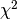# sklearn.datasets.make_gaussian_quantiles¶

sklearn.datasets.make_gaussian_quantiles(mean=None, cov=1.0, n_samples=100, n_features=2, n_classes=3, shuffle=True, random_state=None)[源代码]

Generate isotropic Gaussian and label samples by quantile

This classification dataset is constructed by taking a multi-dimensional standard normal distribution and defining classes separated by nested concentric multi-dimensional spheres such that roughly equal numbers of samples are in each class (quantiles of thedistribution).

Read more in the User Guide.

Parameters: mean : array of shape [n_features], optional (default=None) The mean of the multi-dimensional normal distribution. If None then use the origin (0, 0, ...). cov : float, optional (default=1.) The covariance matrix will be this value times the unit matrix. This dataset only produces symmetric normal distributions. n_samples : int, optional (default=100) The total number of points equally divided among classes. n_features : int, optional (default=2) The number of features for each sample. n_classes : int, optional (default=3) The number of classes shuffle : boolean, optional (default=True) Shuffle the samples. random_state : int, RandomState instance or None, optional (default=None) If int, random_state is the seed used by the random number generator; If RandomState instance, random_state is the random number generator; If None, the random number generator is the RandomState instance used by np.random. X : array of shape [n_samples, n_features] The generated samples. y : array of shape [n_samples] The integer labels for quantile membership of each sample.

Notes

The dataset is from Zhu et al .

References

 [R8] Zhu, H. Zou, S. Rosset, T. Hastie, “Multi-class AdaBoost”, 2009.